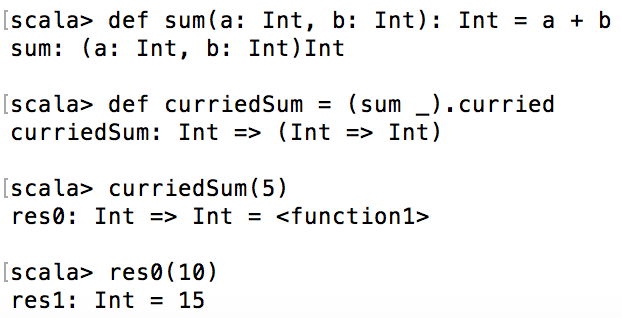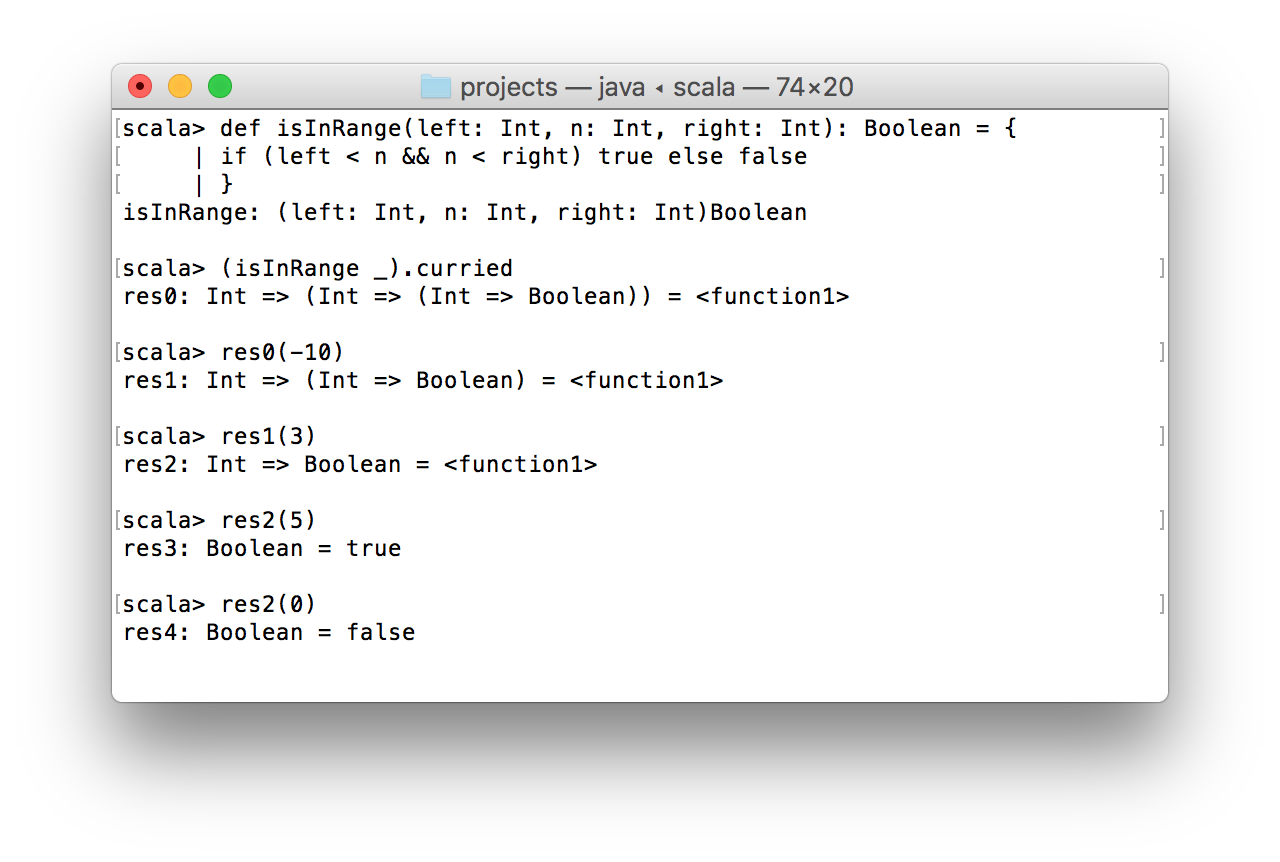# The Difference Between Currying and Partially Applied Functions

### Currying and partially applying may seem the same — both turn your functions into ones with fewer arguments. But there's a difference between them.

· Java Zone · Tutorial
Save
14.02K Views

In this article, I want to show a real difference between curried functions and partially applied functions in Scala. This question is pretty common for those developers who started learning Scala without previous experience in functional programming. Moreover, this blog post may be useful even for experienced Scala developers because, based on my experience, I have had an incorrect understanding of the difference between the curried functions and partially applied functions.

So here is the most classic example of misunderstanding in the question of currying and partially applied functions. I wrote that article ~9 months ago. Since then, I've dived deeper into Scala and figured out what a curried function is and what a partially applied function is.

## Definitions

Before demonstration of code examples, it would be rational to get acquainted with the definitions. For most people, it is enough in order to understand what is the difference.

Currying: decomposition of functions with multiple arguments into a chain of single-argument functions.

Notice, that Scala allows passing a function as an argument to another function.

Partial application of function: pass to a function fewer arguments than it has in its declaration. Scala does not throw an exception when you provide fewer arguments to the function, it simply applies them and returns a new function with rest of arguments that need to be passed.

In general, you can stop reading this article if everything is clear. Otherwise, I recommend looking at some examples for each of function types.

## Currying Examples

Let’s start from the most trivial example of a curried function:

``````def sum(a: Int, b: Int): Int = a + b
//(Int, Int) => Int

def curriedSum = (sum _).curried
//Int => (Int => Int)

curriedSum(5)
//Int => Int

res0(10)
//Int = 15``````

What happened in the code snippet above? At first, I declared the function `sum`. It has two arguments of the `Int` type. Its returning type is `Int` as well.

Then, I create a curried version of the `sum` function. The `curriedSum` function accepts a single argument of the `Int` type and returns a function of the `Int => Int` type.

When I pass 5 into the `curriedSum` , I receive back a new function `res0: Int => Int` (this sample was performed in REPL).

Finally, invoking `res0` with any `Int` parameter we will add this parameter to 5.By invoking a `curried` method on any function of type `FunctionN` where N > 1, we receive a curried version of the function.

Here is another example of currying:

``````def isInRange(left: Int, n: Int, right: Int): Boolean = {
if (left < n && n < right) true else false
}

(isInRange _).curried
//Int => (Int => (Int => Boolean))``````

The logic of the `isInRange` function is pretty simple: If argument `n` is more than `left` but less than `right`, the function returns `true`. Otherwise, it returns `false`.

After we made a currying version of `isInRange`, we can perform the following operations with it:In this screenshot, you can see how we pass arguments one by one and, as a result, we see two boolean results in `res3` and `res4`.

## Partial Application Examples

Now let’s see how partially applied functions look likein practice. I’m going to use the `isInRange` function from the previous section.

``````def isInRange(left: Int, n: Int, right: Int): Boolean = {
if (left < n && n < right) true else false
}

def is5InRange = isInRange(_: Int, 5, _: Int)
//(Int, Int) => Boolean

is5InRange(0, 8)
//true

def between0and10 = isInRange(0, _: Int, 10)
//Int => Boolean

between0and10(5)
//true

between0and10(100)
//false``````

So what happened in the code snippet above? I used `isInRange` as a base for two new functions: `is5InRange` and `between0and10`. Notice, that for missed parameters, you can use an underscore.

Partial application is extremely helpful when you want to create a set of different functions on top of one particular function. In the same way, as I create `between0and10`, it’s easy to create `between50and75` , for instance.

## Summary

So after the theory and practice part, I hope you understood how curried functions differ from partially applied functions. Once again: currying – transformation of a function with multiple arguments into a chain of single-argument functions. Partially applied function – a function that was called with a fewer number of arguments.

Topics:
scala, currying, partially applied functions, tutorial, java

Published at DZone with permission of Alexey Zvolinskiy, DZone MVB.

Opinions expressed by DZone contributors are their own.